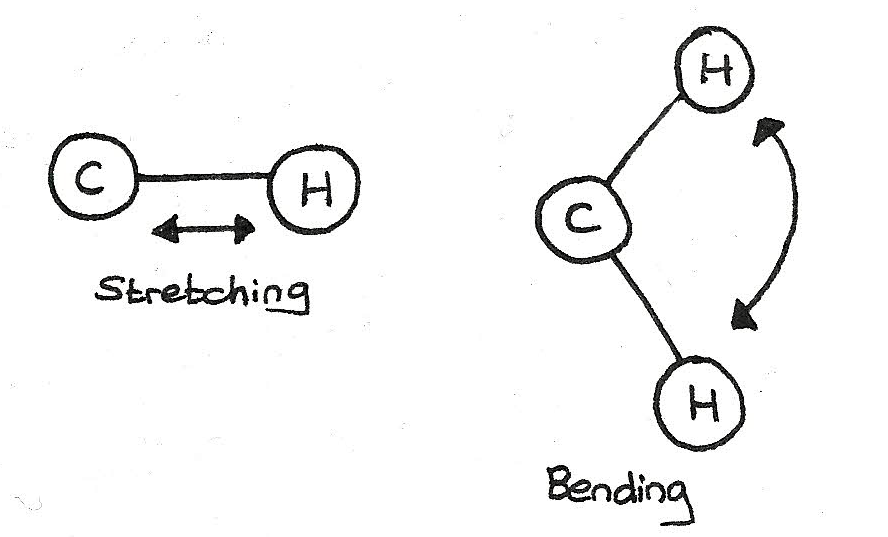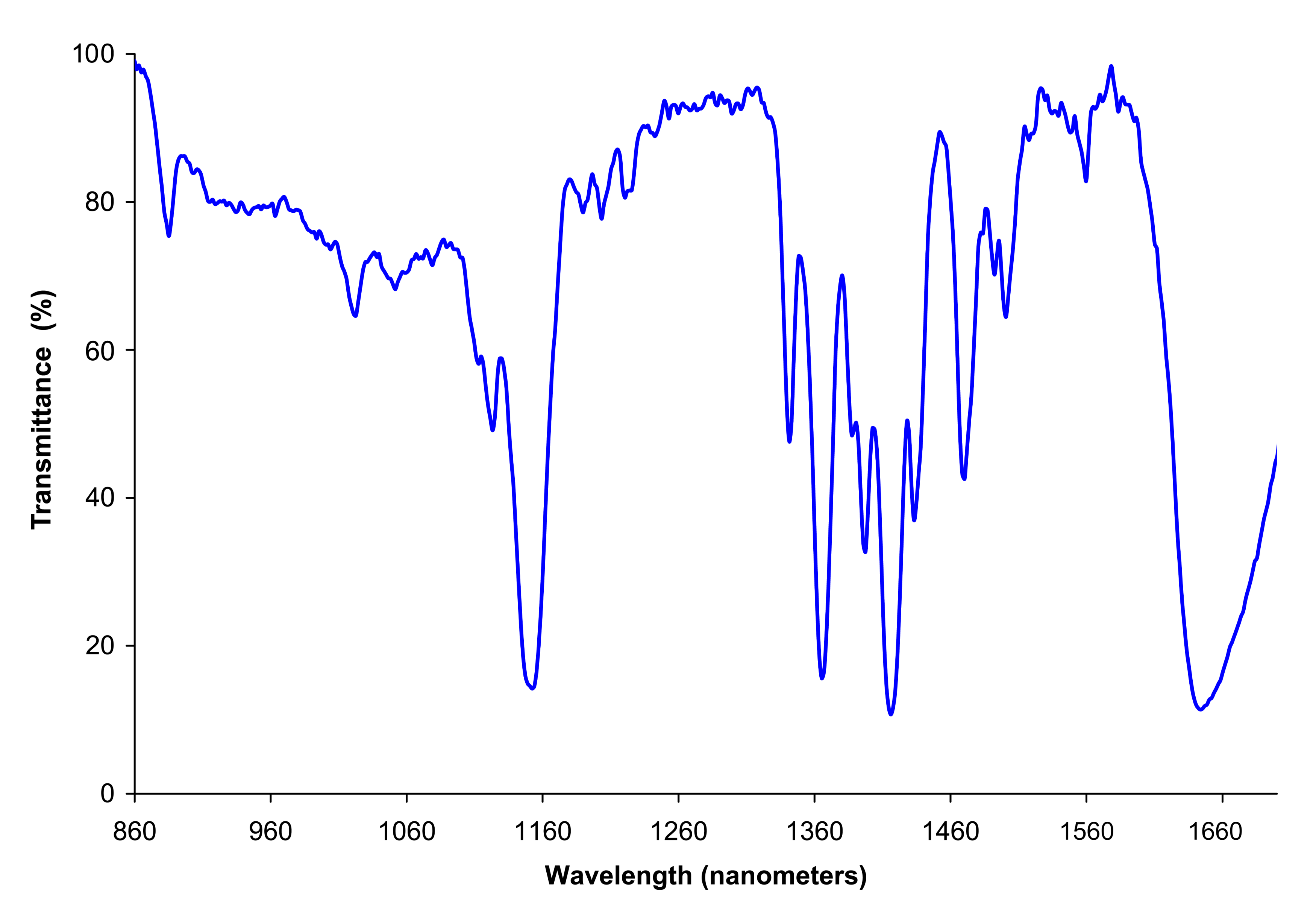# Analysis Techniques

## IR Spectroscopy

All molecules absorb infrared radiation. This absorbed energy makes bonds vibrate through stretching or bending.Every bond vibrates at its own unique frequency, usually between $300$ and $4000cm^{-1}$, the infrared part of the electromagnetic spectrum. The amount of vibration depends on:

• The strength of the bond
• The bond length
• The mass of each atom involved in the bond

These absorptions can be displayed using an infrared spectrum. The spectrum indicates the presence of functional groups in the compound. The peaks (which appear as troughs) represent the absorption of energy at a particular frequency.This technique of detecting the presence of functional groups is used extensively in forensic science such as analysing paint fragments. Other uses include:

• Monitoring the degree of unsaturation in polymers
• Breathalysers for testing for alcohol
• Analysis of drugs

### Identification of functional groups

Using the infrared spectrum, the functional groups can be identified:

Bond Functional Group Wavenumber ($cm^{-1}$)
$C-O$ Alcohols, esters, carboxylic acids 1000-1300
$C=O$ Aldehyde, ketones, carboxylic acids, esters, amides 1640-1750
$C-H$ All organic molecules 2850-3100
$O-H$ Carboxylic acids 2500-3300 (very broad)
$N-H$ Amines, amides 3200-3500
$O-H$ Alcohols, phenols 3200-3550 (broad)​

## Mass Spectrometry

Mass spectrometry is a process where the mass of a molecule is determined by measuring the mass-to-charge ratio of ions. This is useful in order to:

• Monitor breathing during operations.
• Detecting banned substances.
• Analysing molecules in space.

By doing a mass spectrum, the molecular mass, structural features and abundance of isotopes can be determined.

### How mass spectrometry works

When an organic molecule is inserted into a mass spectrometer, some molecules lose an electron and are ionised. The resulting positive ion is called the molecular ion and has the $M^{+}$ symbol.

$$C_{2}H_{5}OH \rightarrow C_{2}H_{5}OH^{+} + 2e^{-}$$

In mass spectroscopy, certain assumptions are made:

• The mass of the lost electron is negligible.
• The molecular ion has a mass equal to the molecular mass of the compound.

During the process, excess energy can cause the molecular ion to vibrate, weakening the bonds. This results in fragmentation of the ion into a fragment ion and natural species. For example:

$$C_{2}H_{5}OH^{+} \rightarrow CH_{3} + CH_{2}OH^{+}$$

### Fragmentation

Fragmentation is the process of causing a positive ion to split into pieces, one of which is a positive fragment and the other a natural species.

Fragment ions are often broken up into further fragments which are detected. The molecular ion, $M^{+}$ produces the peak with the highest m/z value in the mass spectrum.

These produced fragments give information about the structure of a compound. By looking at these fragments, the structure can be found:

m/z value Possible fragment identity
15 $CH_{3}^{+}$
29 $C_{2}H_{5}^{+}$
47 $C_{3}H_{7}^{+}$